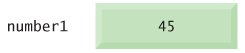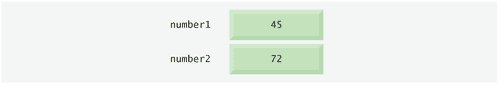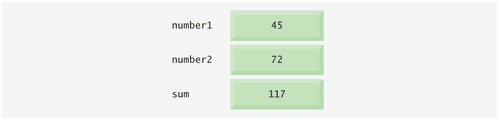# Memory Concepts

Variable names such as number1, number2 and sum actually correspond to locations in the computer's memory. Every variable has a name, a type, a size and a value.

In the addition program of Fig. 2.5, when the statement

```std::cin >> number1; // read first integer from user into number1
```

in line 14 is executed, the characters typed by the user are converted to an integer that is placed into a memory location to which the name number1 has been assigned by the C++ compiler. Suppose the user enters the number 45 as the value for number1. The computer will place 45 into location number1, as shown in Fig. 2.6.

Figure 2.6. Memory location showing the name and value of variable number1.Whenever a value is placed in a memory location, the value overwrites the previous value in that location; thus, placing a new value into a memory location is said to be destructive.

Returning to our addition program, when the statement

```std::cin >> number2; // read second integer from user into number2
```

in line 17 is executed, suppose the user enters the value 72. This value is placed into location number2, and memory appears as in Fig. 2.7. Note that these locations are not necessarily adjacent in memory.

Figure 2.7. Memory locations after storing values for number1 and number2.Once the program has obtained values for number1 and number2, it adds these values and places the sum into variable sum. The statement

```sum = number1 + number2; // add the numbers; store result in sum
```

that performs the addition also replaces whatever value was stored in sum. This occurs when the calculated sum of number1 and number2 is placed into location sum (without regard to what value may already be in sum; that value is lost). After sum is calculated, memory appears as in Fig. 2.8. Note that the values of number1 and number2 appear exactly as they did before they were used in the calculation of sum. These values were used, but not destroyed, as the computer performed the calculation. Thus, when a value is read out of a memory location, the process is nondestructive.

Figure 2.8. Memory locations after calculating and storing the sum of number1 and number2.C++ How to Program (5th Edition)
ISBN: 0131857576
EAN: 2147483647
Year: 2004
Pages: 627